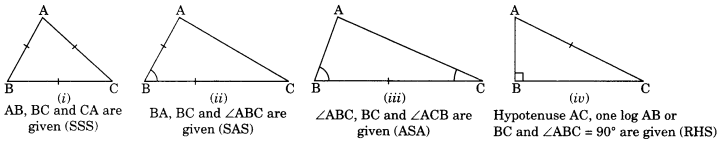On this page, you will find Practical Geometry Class 7 Notes Maths Chapter 10 Pdf free download. CBSE NCERT Class 7 Maths Notes Chapter 10 Practical Geometry will seemingly help them to revise the important concepts in less time.

## CBSE Class 7 Maths Chapter 10 Notes Practical Geometry

### Practical Geometry Class 7 Notes Conceptual Facts

1. By using properties of a transversal and parallel lines, a line parallel to a given line passing through a given point lying not on the line can be drawn.
Let P is any point outside of the given line T. P is joined to any point Q on the line T.
Join P and Q. Draw an angle 2 equal to angle 1. We get m || l.

2. We can draw a triangle if any one of the following conditions are given:

• Three sides (By SSS criterion)
• Two sides and the angle between them (By SAS criterion)
• Two angles and the side included between them (By ASA criterion)
• The hypotenuse and a leg of a right-angled triangle (By RHS criterion)